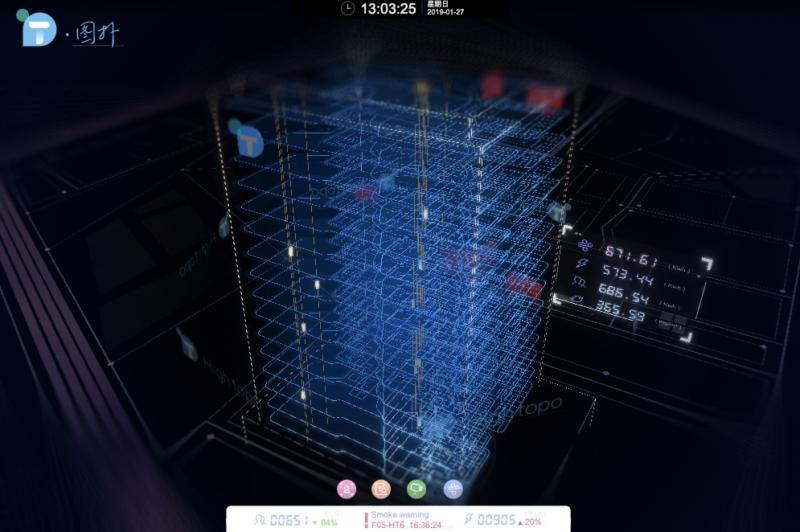## 前言

• 智慧化 -- 智慧楼宇是一个生态系统，像人一样拥有感知能力、自我判断能力以及控制能力。
• 绿色化 -- 绿色建筑在耗能、产能以及能源管理方面实现绿色化，楼宇安防实现绿色化监控。
• 运行成本可控制 -- 基于可持续发展的要求，现代建筑、商业建筑需运行50年以上，建筑在运行过程中能源消耗巨大，如何降低运营成本降低，使建筑在低碳、环保的状态下健康运行。

## 界面简介及效果预览

### 监控界面效果

• 人员进入大厦的实时监控，面板中动态刷新人员进入大厦的头像以及当前大厦人数等实时信息。
• 大厦电梯运行情况实时监控，系统中展示电梯当前的运行位置以及是否在运行等信息。
• 大厦某个具体楼层监控数据的实时监控，通过柱状图的形式展示了当前楼层具体信息的大小。
• 大厦管道的实时监控，展示了当前智能建筑所有管道的运行情况。

## 场景关键动画代码分析

### 1. 地图路线动画代码分析

``````  // currentIndex 为当前路径绘制到的点的索引
// points 为当前路径所有点的信息  currentPoints 为绘制过程中点的信息
// segments 为当前路径所有点的连接方式信息 currentSegments 为绘制过程中点的连接方式信息

// 即上述此时 A 点信息
let fromPoint = points[currentIndex];
// 即上述此时 B 点信息
let toPoint = points[currentIndex + 1];
// 通过 AB 两点信息组成一条 AB 方向的向量
let pointVector = new ht.Math.Vector2(toPoint.x - fromPoint.x, toPoint.y - fromPoint.y);
// 记录该向量的长度，用于判断 AC 是否大于等于 AB
let pointVectorLength = pointVector.length();

let currentPoints = [], currentSegments = [];

for(let i = 0; i < currentIndex + 1; i++) {
currentPoints.push({
x: points[i].x,
y: points[i].y
});
currentSegments.push(segments[i]);
}``````

``````  // addLength 为每次增加的线段长度值，该程序中使用 500 即每次长度增加 500
let nextVectorLength = currentVectorLength + addLength,
tempPoint;

// 判断 AC 线段的长度是否大于 AB
if(nextVectorLength > pointVectorLength) {
nextVectorLength = pointVectorLength;
}

pointVector.setLength(nextVectorLength);

// 即为 C 点坐标
tempPoint = {x: pointVector.x + fromPoint.x, y: pointVector.y + fromPoint.y};
// 往 currentPoints 添加 C 点坐标
currentPoints.push(tempPoint);
// 往 currentSegments 添加 C 点连接方式，此程序中都为直线连接，所以值都为 2
currentSegments.push(2);
// roadNode 即为 ht.Shape 类 重新设置 ht.Shape 类点的信息
// 重新设置 ht.Shape 类点的连接信息

### 2. 水波以及扫描动画代码分析

`````` // waterWaveNodes 所有水波节点
interval: 100, // 指每隔 100 ms 调用 action 函数一次
action: function(data){
// 判断 waterWaveNodes 是包含 data
if(waterWaveNodes.indexOf(data) > -1) {
// 获取此时图标矩形框信息 circleRect 是个长度为 4 的数组 分别表示 x, y, width, height
let circleRect = data.a('circleRect');

if(circleRect) {
// 通过修改高度来变大水波大小
let nextHeight = circleRect + 10;

// 高度最大值为 250
if(nextHeight < 250) {
// 对应修改 y 的大小，y 的增加大小为高度的一半
circleRect = circleRect - 5;
circleRect = nextHeight;
data.a('circleRect', circleRect);
data.a('borderColor', 'rgba(255, 133, 133, ' + (1 - circleRect / 250) + ')');
}
else {
data.a('circleRect', [-0.5,128,257,0]);
data.a('borderColor', 'rgba(255, 133, 133)');
}

}
else {
data.a('circleRect', [-0.5,128,257,0]);
}

}
}
};

### 3. 数字变化动画代码分析

``````  // numNode(1-7) 为 2d 面板中对应数字的节点
// data.a('ht.value', number) 即为动态修改 attr 上的 ht.value 信息，之后图纸会自动更新新赋予的数值
// getRandomValue 为自己封装的产生随机数的方法
interval: 1000,
action: (data) => {
if(data === numNode1 || data === numNode2) {
data.a('ht.value', util.getRandomValue([500, 999], 5));
}
if(data === numNode3 || data === numNode4) {
data.s('text', util.getRandomValue([0, 30], 2) + '%');
}
if(data === numNode5) {
data.a('ht.value', util.getRandomValue([0, 99999], 5, 3));
}
if(data === numNode6) {
data.a('ht.value', util.getRandomValue([0, 100], 2));
}
if(data === numNode7) {
data.a('ht.value', util.getRandomValue([0, 100], 2));
}
}
};

### 4. 柱状图高度动画代码分析

`````` charts.forEach((chart) => {
!chart.a('tall') && chart.a('tall', chart.getTall()); // 将高度存储到 attr 上
chart.setTall(0); // 设置初始高度为 0
});
interval: 25,
action: function(data){
if(charts.indexOf(data) > -1) {
if(finishNum !== chartLength) {
if(data.getTall() !== data.a('tall')) {
let nextTall = data.getTall() + deep; // deep 为每次增加的高度
let tall = data.a('tall'); // 获取初始化高度
// 判断下一个高度是否大于初始化高度
if(nextTall < tall) {
data.setTall(nextTall);
}
else {
data.setTall(tall);
finishNum++;
}
}
}
}
}
};

### 5. 3d 镜头推进代码分析

3d 场景中视野的推进后退都是通过 HT api 提供的修改 eye 以及 center 的数值方法来实现，通过不断调用 setEye 以及 setCenter 方法来达到修改视角的目的，eye 类比人眼睛所处的位置，center 类比人眼睛聚焦的位置，以下为实现镜头推进关键的伪代码：

`````` let e = ht.Default.clone(g3d.getEye()), // 获取当前眼睛的位置
c = ht.Default.clone(g3d.getCenter()); // 获取当前眼睛聚焦的位置
// eye 为需要修改的对应 eye 值
// center 为需要修改的对应 center 值
// 以下为分别获取 eye 与 center 在 xyz 三个坐标轴之间的差值
let edx = eye - e,
edz = eye - e,
edy = eye - e,
cdx = center - c,
cdz = center - c,
cdy = center - c;
// 开启不断修改 eye 与 center 的动画
ht.Default.startAnim({
duration: time ? time : 3000,
easing: function(t){ return t; },
finishFunc: function() {
if(typeof cb === 'function') {
cb();
}
},
action: function (v) {
// v 为从 0-1 变换的值
g3d.setEye([
e + edx * v,
e + edz * v,
e + edy * v
]);
g3d.setCenter([
c + cdx * v,
c + cdz * v,
c + cdy * v
]);
}
});``````